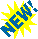## Flatness of the rotation curves of the galaxies

### Exit the recourse to a massive halo

Here the summary, in English of a paper, presenting a work made with D. Méra (Cral, ENS Lyon) and J.-B. Baillon (IGD):
We use a new original method to calculate the surface density of a galaxy from its rotation curve assuming that all the mass is in the galactic plane. We show that this method gives accurate masses for the disk. Moreover, given a rotation curve for r less than Rg only (Rg is the galaxy radius), we find that the surface density is nearly uniquely determined except near the edge of the disk. We also demonstrate that the common assumption that a flat rotation curve leads to a density profile Sigma(R) as 1/R is false for a finite disk. We derive for the MilkyWay a mass of 1.4 10^11 solar mass. The exponential profile is well reproduced with a scale length between 3.6 and 5 kpc. The local surface density is found to be 125 +/- 10 solar mass/pc^2, compatible with other independant determinations.
Click here to obtain the paper (1996). A new presentation (2007) and a chapter a book (en français) about the problem of the dark matter (2003). Program (2014) with maple18.
Update, july 2017 new program and many references.

###Presentation :
The rotation curves of galaxies, such as they are observed, remain flat to large distances, say from 10 to 15 kpc center of a usual spiral galaxy which one studies the disk speeds of the spiral arms. This fact raises a rather crucial question, and the only explanation given for the moment, is to admit the existence of a massive spherical halo of matter including such a galaxy. This problem constituted a enigma opening the way with an active research of " hidden mass" in the universe.
We show that:
i) Within a Newtonian framework, the phenomenon of flatness of the curves is very simply explained without recourse to a possible massive halo and the proof shows why there is in fact less " hidden mass ".
ii) The Einsteinian study of these curves changes the manner of tackling this problem of hidden mass coming from rotation curves of the galaxies. This can seem surprising, insofar as the gravitational field created by a galaxy is very weak, but on such a scale the Einsteinian correction with the usual Newtonian gravitation proves to be non negligeable.

###On the existing methods to study the rotation curves :
An excellent presentation of the three existing methods are explained in the work of Binney and Tremaine entitled " Galactic Dynamics ". These three methods have as names, the method of the elliptic integrals (based on the disc seen like rings of matter), the method of the Bessel transform (based on asymptotic properties of the gravitational potential created by the disc of matter) and finally the method of the sphéroides, most used (based on the disc seen like limit of thin ellipsoids). The common point of these three methods is the fact that, on the basis of a surface density, the disk speed is expressed by a double integral which is not absolutely convergent. Thus these three methods deducing a rotation curve from a surface density (presumedly known) do not make it possible to solve the initial problem which is to deduce the distribution of masses starting from the observed rotation curve (except in academic cases by knowledge of indefinite integrals for example).
Our direct method; comparison with the preceding ones:
It rests on the simulation of a spiral galaxy by a disc of radius R made of N massive bodies distributed with an axial symmetry. Taking into account that these bodies turn while following a curve of rotation given, then the balance of the radial forces between N body leads to a set of equations: it is a linear system (of which the unknown factors are the masses of N body) that one reverses and which thus provides the surface density curve. In fact it is a " inverse method of the N-bodies problem", which give results very precise and easy to implement (the curves presented rest on a simulation by 40 000 points of a galactic disc).
Our method is a riemanian approximation of the double integral coming from the method which uses the elliptic integrals, it is thus theoretically equivalent to it. To establish equivalence between the method of the Bessel transform and that of the elliptic integrals, I have permuted the integrals and introduces principal values (the double integrals being not absolutely convergent), then to transform Bessel functions on the one hand and elliptic integrals on the other hand into hypergeometric series. Our method thus gives theoretically the same results as the method of the elliptic integrals and than that of the Bessel transform. As this last method is theoretically invertible, our method provides a surface density whatever the curve of rotation given, and this
##### without recourse to a massive halo.
It is obviously always possible to add a light halo, our method spreads without sorrow. But the results are different from those coming from the usually used method, that of the thin ellipsoids (which implies the need for a massive halo). Cf. "Towars a consistent model of the galaxy : Derivation of the model"; A. & A. vol 330, 1998, from Mera, Chabrier and Schaeffer.

###: this page was writtened in 1997, but now (februar 2006) two papers (using Bessel functions) obtain the same results (without the need of a massive halo) :
Burkhard Fuchs, Asmus Boehm, Claus Moellenhoff, Bodo L. Ziegler : Quantitative interpretation of the rotation curves of spiral galaxies at redshifts z~0.7 and z~1.
F.I. Cooperstock, S. Tieu : Perspectives on Galactic Dynamics via General Relativity.